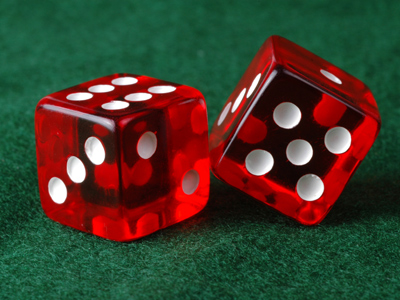A solid shape is one that has volume, such as a cube.

# Shapes (Very Easy)

Shapes that do not have volume (they are flat) are called two-dimensional (2D), e.g. the square and the circle. How many more two-dimensional shapes can you think of?

We have four sections of 11-plus Maths quizzes on shapes (Very Easy, Easy, Medium and Difficult) and this is our first and easiest. You should get all of these questions correct, so make sure you do before you move onto our next quiz. The third section (Medium) focuses on triangles and our hardest ones takes a look at quadrilaterals.

Do you have a favourite shape? Have you ever tried to draw an animal using just one or two shapes? It's fun! See if you can draw a pig using only circles. But before you get your pencils out, play this quiz.

TIP! A quadrilateral is a closed 2D-shape formed by four straight lines, e.g. a square.

1.
What is the name of the object that is being described?
It's a closed 3D-shape that has six identical square sides, where each square side is at right angles to the other square sides that it joins with at its edges.
Tetrahedron
Cube
Cylindrical prism
Pyramid
The cube and the sphere are probably the commonest 3D shapes
2.
What is the name of the object that is being described?
It's a closed 3D-shape that is formed by rotating a circle.
Sphere
Torus
Ellipsoid
Oblate spheroid
If you are really interested, look up the other words in this question.The shape of the Earth is very close to that of an oblate spheroid
3.
What is the name of the object that is being described?
It's a small dot.
Spot
Place
Point
Location
In maths, a 'point' is used to locate or position something
4.
What is the name of the object that is being described?
It's a closed quadrilateral with two pairs of parallel sides which are not joined at right angles to each other and which are not all identical in length.
Parallelogram
Square
Rhombus
Rectangle
It's not a rectangle because its parallel sides are joined at right angles to each other
5.
What is the name of the object that is being described?
It's a closed 2D-shape with three straight sides that are joined up at their ends.
Square
Pyramid
Triangle
Cube
This is the ONLY three-sided shape
6.
What is the name of the object that is being described?
It describes a shape that has volume.
Bulk
Size
Dimension
Solid
In maths it's called a 'solid' shape. Did you know that 'solid geometry' is concerned with the study of 3D-space?
7.
What is the name of the object that is being described?
It's a closed five-sided 2D-shape, the sides of which are equal in length and joined at the same angle to each other.
Regular hexagon
Regular pentagon
Regular heptagon
Regular octagon
If the sides weren't all the same length, it would be called an 'irregular' pentagon. Polygons are closed shapes that have three or more straight sides: hexagon (6 sides), heptagon (7 sides), octagon (8 sides), nonagon (9 sides), decagon (10 sides). LEARN this stuff!
8.
What is the name of the object that is being described?
It's a closed quadrilateral, the sides of which are equal in length and joined at right angles to each other.
Square
Rectangle
Parallelogram
Rhombus
9.
What is the name of the object that is being described?
It's a measure of the amount of rotation (turn).
Spin
Twist
Swivel
Angle
In maths it's called 'angle' NOT 'angel'. God knows why!
10.
What is the name of the object that is being described?
It's a closed 2D-shape in the form of a perfect hollow ring.
Oval
Diamond
Circle
Rhombus
The points on a circle are all at the same distance from its centre
Author:  Frank Evans